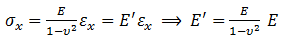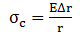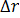#### Chocking Model

This model is basis of Kellogg’s method which has been used for local stresses calculation for many years in piping engineering field. As shown in figure 1, a cylinder which is completely symmetric in its longitudinal axis is under a radial symmetric and uniform load on its shells. The purpose of this section is calculation of longitudinal and circumferential local stresses (discontinuity stresses) in its discontinuity area.In infinite beam calculations, it is assumed the beam lateral faces can move freely. But referring to the strip shown in figure 2, the lateral forces are restricted in circumferential direction. So, by applying Hook’s law:

For developing a method to calculate the local stresses, a strip of element has been assumed as shown in Figure 2. If it is proved that, for the strip, support load is proportional to radial deformation; the infinite beam on an elastic deformation model can be used for simulation of the strip.Figure 2: Loads on a strip of element

Referring to Fig 2, the support load       causes a circumferential force, named as N, which creates a circumferential membrane stress on its surface.(1)

With substituting            in equation (1):(2)

From applying Hook’s law in circumferential direction:(3)

(4)

With equations (3) and (4):(5)

From equations (2) and (5):(6)

Equation (6) can be written as:(7)

Equation (7) shows the support load is proportional to radial displacement. So the strip behavior is similar to a beam on an elastic foundation. For using equations (18) to (21) mentioned in “Governed differential equation for a beam on an elastic foundation”, β should be calculated based on the chocking model parameters.

This kind of stress is in longitudinal direction and caused by a bending moment. So, regarding ASME BPVC Section VIII div 2, it is placed in bending stress category. (Refer to figure 3)

1- β Calculation(8)

(9)

With (8) and (9):(10)

It is obvious that E' must be used as elastic modulus in equation (11) mentioned in “Governed differential equation for a beam on an elastic foundation”. Moreover for calculation of β, k should be extracted based on chocking model parameters too as follows:(11)

From (11) mentioned in “Governed differential equation for a beam on an elastic foundation”, (10) and (11):(12)

2- Effective Discontinuity Area Calculation

Radius of discontinuity area is important because pad length is selected based on that. Referring to Fig. 5.3 of , shear force decreases to 20% of the concentrated force at                           .If this length increases to                        the bending moment decreases to 10% of its value at support load.3-Local Stress (Discontinuity Stress) Calculation:

There are three types of local stresses as mentioned bellow:

3-1- Longitudinal Bending Stress(13)

M is calculated from equations (20) mentioned in “Governed differential equation for a beam on an elastic foundation” at  x=0.  So,(14)

With (13) and (14):(15)

3-2-Circumferential membrane Stress

This local stress is uniformly applied in circumferential direction and considered as a membrane stress Figure 3).(16)

With equations (5) and (16):(17)

can be calculated by equation (18) mentioned in “Governed differential equation for a beam on an elastic foundation” at x=0 :(18)

With equations (5) and (16):(19)

3-3-Circumferential bending Stress

Because of poison effect, the longitudinal stress calculated by equation (15) causes a displacement in circumferential direction. This displacement is not a free one because of resistance against it. Therefore a bending circumferential stress, as shown in figure 3, is developed. Its value is insignificant comparing to     .Figure 3: Local stresses developed in chocking model(20)